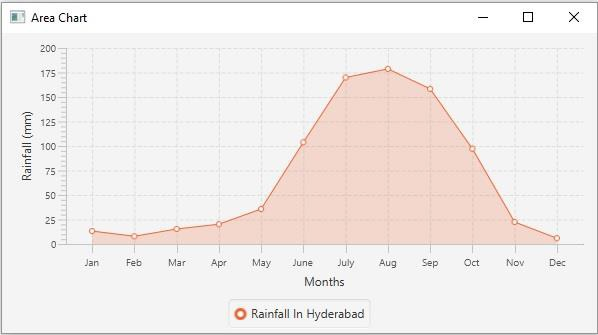# How to create an area chart using JavaFX?

The area chart accepts a series of data points (x, y) as input values, connects them using a line, and maps the area between the obtained line and the axis. In JavaFX, you can create an area chart by instantiating the javafx.scene.chart.AreaChart class.

While instantiating this class you must pass the two objects of the Axis class representing the x and y-axis (as parameters of the constructor). Since the Axis class is abstract you need to pass objects of its concrete subclasses, NumberAxis (for numerical values) or, CategoryAxis (String values).

Once you create the axes you can set labels to them using the setLabel() method.

## Setting data

The XYChart.Series represents the series of data items. You can create a series of points for the line by instantiating this class. This class contains an observable list that holds all the points in the series.

The XYChart.Data represents a specific data point in the x-y plane. To create a point, you need to instantiate this class by passing the x and y values of the particular point.

Therefore, to create data for a line −

• Create a required number of points by instantiating the XYChart.Data class.

• Create a series by instantiating the XYChart.Series class.

• Get the observable list of the XYChart.Series class using the getData() method.

• Add the created data series to the area chart as −

areachart.getData().add(series);

## Example

import javafx.application.Application;
import javafx.scene.Scene;
import javafx.scene.chart.AreaChart;
import javafx.stage.Stage;
import javafx.scene.chart.NumberAxis;
import javafx.scene.chart.XYChart;
import javafx.scene.layout.StackPane;
public class AreaChartExample extends Application {
public void start(Stage stage) {
//Defining the X and Y axes
NumberAxis xAxis = new NumberAxis();
NumberAxis yAxis = new NumberAxis(); …
//Setting labels to the axes
xAxis.setLabel("Months");
yAxis.setLabel("Rainfall (mm)");
//Creating the Area chart
AreaChart<String, Number> areaChart = new AreaChart(xAxis, yAxis);
//Prepare data for the area chart
XYChart.Series series = new XYChart.Series();
//Setting the name to the line (series)
//Setting data to the area chart
//Creating a stack pane to hold the chart
StackPane pane = new StackPane(areaChart);
//Setting the Scene
Scene scene = new Scene(pane, 595, 300);
stage.setTitle("Area Chart");
stage.setScene(scene);
stage.show();
}
public static void main(String args[]){
launch(args);
}
}

## Output